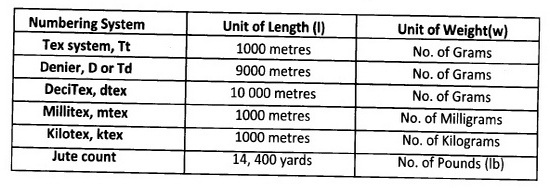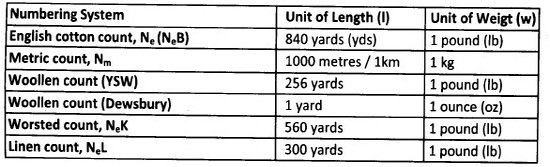### Yarn Numbering System (Yarn Count) | Direct Count System | Indirect Count System

Yarn Count:
Count is a numerical value, which express the coarseness or fineness (diameter) of the yarn and also indicate the relationship between length and weight(the mass per unit length or the length per unit mass)of that yarn. Therefore, the concept of yarn count has been introduced which specifies a certain ratio of length to weight.

The fineness of the yarn is usually expressed in terms of its linear density or count. There are a number of systems and units for expressing yarn fineness. But they are classified as follows .

Types of Yarn Count:

1. Direct Count System
2. Indirect Count System
1. Direct Count System:
The weight of a fixed length of yarn is determined. The weight per unit length is the yarn count! The common features of aII direct count systems are the length of yarn is fixed and the weight of yarn varies according to its fineness.

The following formula is used to calculate the yarn count:

N= (W×l) / L

Where,
N =Yarn count or numbering system
W =Weight of the sample at the official regain in the unit of the system
L=Length of the sample
l=Unit of length of the sampleIn brief, definition of the above Systems is as follows:
1. Tex system ..........................NO. of grams per 1000 meters
2. Denier .................................No. of Grams per 9000 meters
3. Deci Tex ..............................No. of grams per 10,000 metres
4. Millitex ................................No. of milligrams per 1000 metres
5. Kilotex............................... .No. of kilograms per 1000 metres.
6. Jute count........................No. of lb per 14,400 yds
The Tex of a yarn indicates the weight in grammes of 1000 metres yarn. So that 40Tex means 1000 meters of yarn weigh 40gm.

From above discussion it is concluded that, higher the yarn number(count) coarser the yarn and lower the number finer the yarn.

2. Indirect Count System:
The length of a fixed weight of yarn is measured. The length per unit weight is the yarn count. The common features of all indirect count systems are the weight of yarn is fixed and the Length of yarn varies according to its fineness.

The following formula is used to calculate they are count:

N = (L×w) / W×l

Where,
N =Yarn count or numbering system
W =Weight of the sample at the official regain in the unit of the system
L=Length of the sample
l=Unit of length of the sample
w = Unit of weight of the sample.1. Ne: No of 840 yards yarn weighing in One pound

2. Nm: No of one kilometer yarn weighing in One Kilogram

The Ne indicate show many hanks of 840 yards length weigh one English pound. So that 32 Ne Means 32 hanks of 840yards i.e.32x840 yards length weigh one pound.

For the determination of the count of yarn, it is necessary to determine the weight of a known length of the yarn. For taking out known lengths of yarns, a wrap-reel is used. The length of yarn reeled off depends upon the count system used. One of the most important requirements for a spinner is to maintain the average count and count variation within control.

Yarn Count Variation:
The term count variation is generally used to express variation in the weight of a lea and this is expressed as C.V.%. The number of samples and the length being considered for count checking affects this. While assessing count variation, it is very important to test adequate number of leas. After reeling the appropriate length of yarn, the yarn is conditioned in the standard atmosphere for testing before it's weight is determined.

You may also like:
Sharing Knowledge: Students, teachers and professionals can publish your article here. It is a platform to express your knowledge throughout the world. For details: Submit Article

### Founder and Editor:Mazharul Islam Kiron is a textile consultant, entrepreneur, blogger and researcher on online business promotion. He is working as a consultant in several local and international companies. He is also a contributor of Wikipedia.